# Lists in PythonWhat are lists in Python? Lists are one of the six built-in types of sequences in Python programming language. A sequence is the most basic data structure in Python programming language and each element of a sequence has its own index. The first element has the index 0, the second has the index 1 and so forth. There are operations you can perform with all sequence types and there are specific methods only for each sequence. The len() function helps you to find the length of a sequence like shown in Figure 1.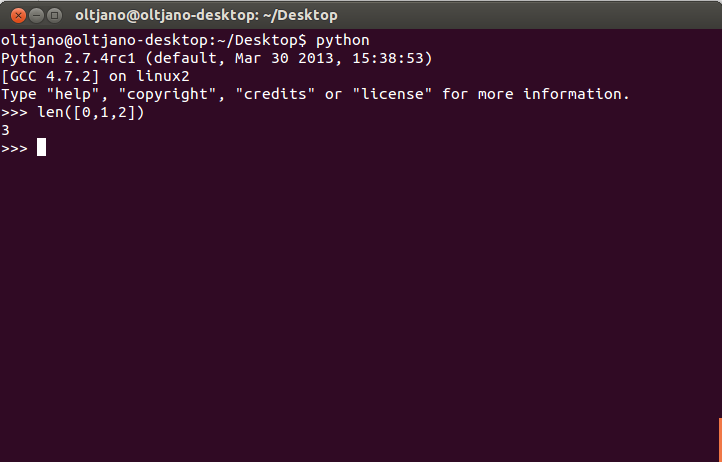Figure 1

Inside the len function is a list with three elements. You can see that a list is constructed by square brackets. If you want to find the largest or the smallest element of the list you can use max() (for maximum), and min() for minimum.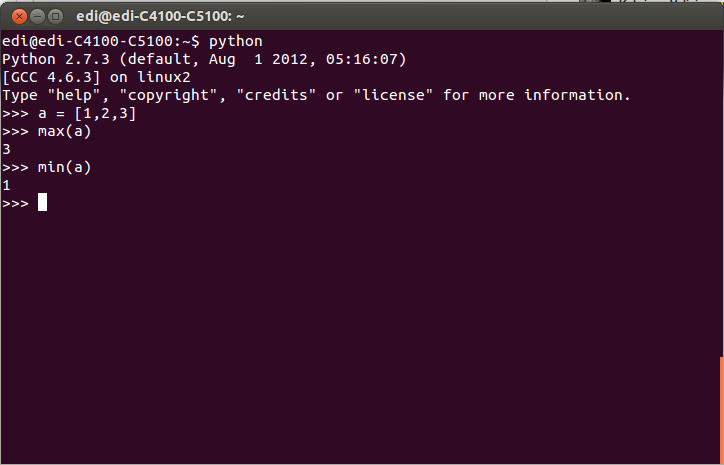Figure 2

Figure 2 shows that we created a list with three elements and assigned it to the a variable. We used the max() and min() function to find the largest and  the smallest elements of the list. Now you know that elements in a list are separated by commas and a list can hold any type of element, strings, integers, tuples other lists etc. It does not matter if  the items in a list are of the same type or not. Now open your terminal and create a list and assign it to the ‘google’ variable like shown in Figure 2.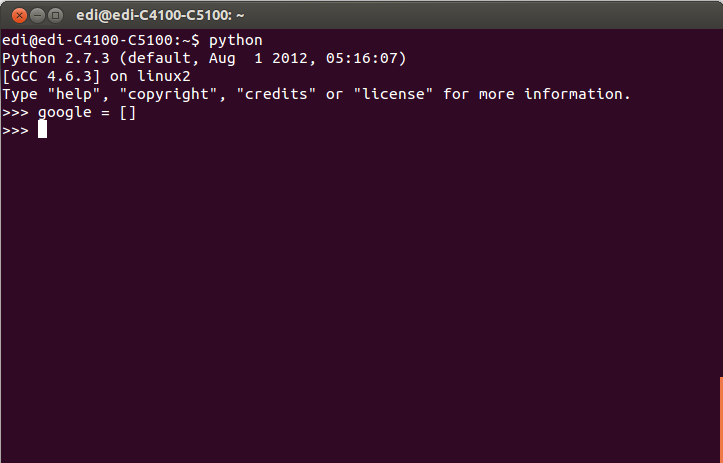Figure 3

Our list is empty and it is time to add some elements to it. We will use the append() method, which is a specific method for lists. I show you how to use the append() method in Figure 4.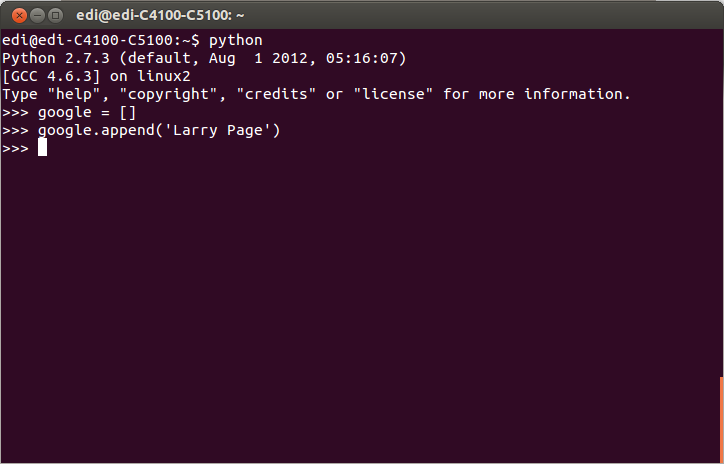Figure 4

To insert an element inside the list with the append() method you put the element you want to append inside the brackets and it is done. You can add as many elements as you want. Time to add more elements with the append() method and find the index for each element on the list. We will use the index() method. This method takes an element of the list as input and finds its index.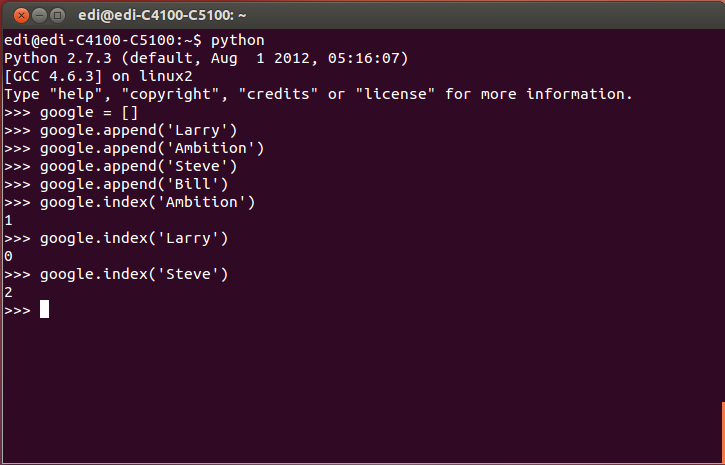Figure 5

We added three new elements on the list, ‘Amibtion’, ‘Larry’ and ‘Steve’. After that we used the index() method to find the index for every element of the list. To find the  index of an element with the index() method you put the element you want to find the index as an input for the index() method. Unixmen, how do I extract elements form a list? To extract elements from a list you can use indexing or slicing. Figure 6 teaches you how to use indexing and Figure 7 teaches you how to use slicing.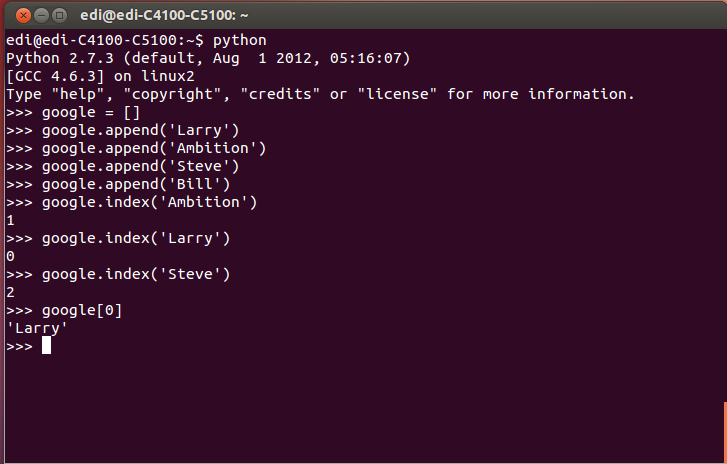Figure 6

We use the name of the list and the index of the element we wanted to extract.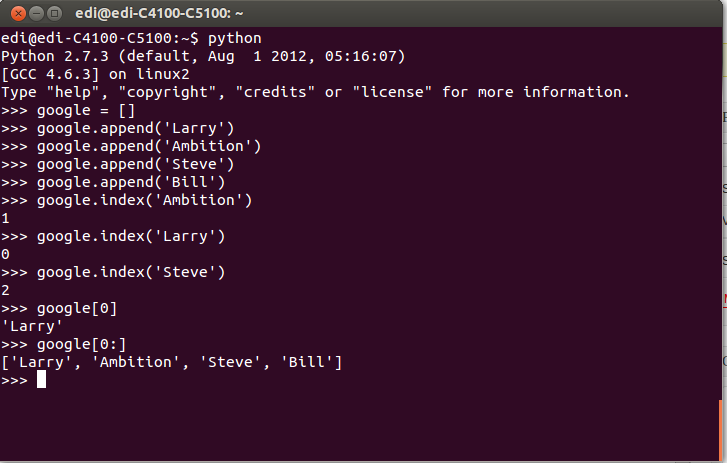Figure 7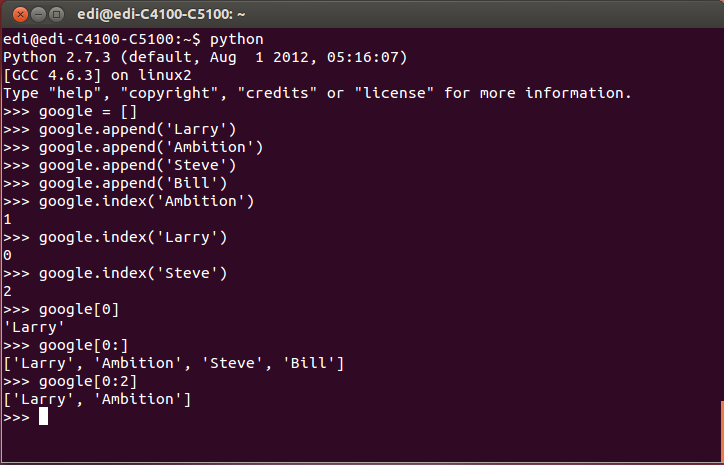Figure 8